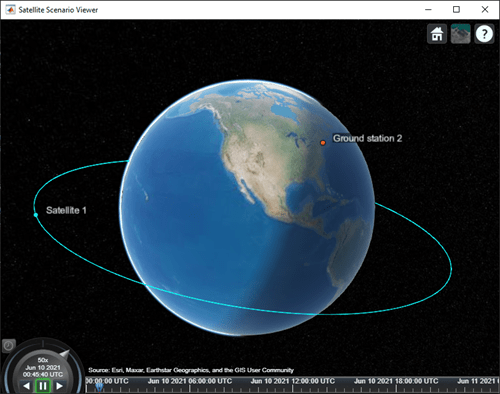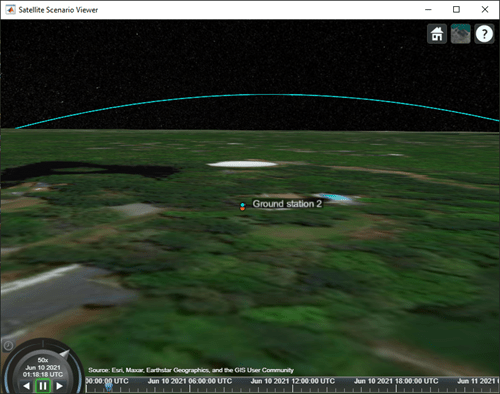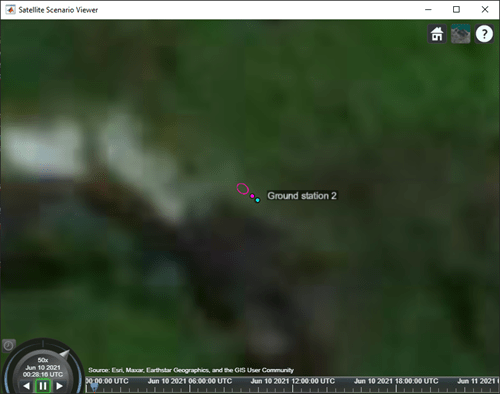# pointAt

Point satellite at target

Since R2021a

## Syntax

``pointAt(sat,coordinates)``
``pointAt(sat,target)``
``pointAt(sat,'nadir')``
``pointAt(sat,attitudetable)``
``pointAt(sat,attitudetable,Name=Value)``
``pointAt(sat,attitudetimeseries)``
``pointAt(sat,attitudetimeseries,Name=Value)``
``pointAt(gimbal,'none')``
``pointAt(gimbal,coordinates)``
``pointAt(gimbal,target)``
``pointAt(gimbal,'nadir')``
``pointAt(gimbal,steeringtable)``
``pointAt(gimbal,steeringtimeseries)``

## Description

### Satellite Object

````pointAt(sat,coordinates)` steers the satellites in the vector `sat` toward the geographical coordinates [latitude; longitude; altitude] specified by `coordinates`.```
````pointAt(sat,target)` steers the satellites specified by `sat` toward the specified `target`. The input `target` can be another satellite or ground station.```
````pointAt(sat,'nadir')` steers the satellites specified by the row vector `sat` toward the nadir direction. `'nadir'` is the default pointing direction.```
````pointAt(sat,attitudetable)` sets the attitude of the satellite `sat` such that it follows the attitudes provided in `attitudetable`, which is a MATLAB® `timetable` object. ```
````pointAt(sat,attitudetable,Name=Value)` specifies options using one or more name-value arguments in addition to the input arguments in the previous `attitudetable` syntax. For example, to interpret the provided attitude values as the rotation from the Geocentric Celestial Reference Frame (GCRF) to the body frame, set `CoordinateFrame` to `inertial`. ```
````pointAt(sat,attitudetimeseries)` sets the attitudes of the satellite `sat` such that it follows the attitude provided in `attitudetimeseries`, which is a MATLAB `timeseries` object. ```
````pointAt(sat,attitudetimeseries,Name=Value)` specifies options using one or more name-value arguments in addition to the input arguments in the previous `attitudetimeseries` syntax. For example, to interpret the provided attitude values as the rotation from the GCRF to the body frame, set `CoordinateFrame` to `inertial`. ```

### Gimbal Object

````pointAt(gimbal,'none')` sets the gimbal angles (gimbal azimuth and gimbal elevation) of the gimbals in the vector `gimbal` to zero. This setting is the default.```
````pointAt(gimbal,coordinates)` steers the gimbals in the vector `gimbal` toward the geographical coordinates [latitude; longitude; altitude] specified by `coordinates`.```

example

````pointAt(gimbal,target)` steers the gimbals in the vector `gimbal` toward the specified `target`.```
````pointAt(gimbal,'nadir')` steers the gimbals specified by the row vector `gimbal` toward the nadir direction of their parents, namely, their parent's latitude, longitude, and 0 meter altitude.```
````pointAt(gimbal,steeringtable)` sets the orientation of the gimbals to align with the azimuth and elevation angles provided in `steeringtable`, which is a MATLAB `timetable` object. ```
````pointAt(gimbal,steeringtimeseries)` sets the orientation of the gimbals to align with the azimuth and elevation angles provided in `steeringtimeseries`, which is MATLAB `timeseries` object. ```

## Examples

collapse all

Create a satellite scenario object.

```startTime = datetime(2021,6,10); % 10 June 2021, 12:00 AM UTC stopTime = datetime(2021,6,11); % 11 June 2021, 12:00 AM UTC sampleTime = 60; % seconds sc = satelliteScenario(startTime,stopTime,sampleTime);```

Add a satellite to the scenario.

```semiMajorAxis = 10000000; % meters eccentricity = 0; inclination = 10; % degrees rightAscensionOfAscendingNode = 0; % degrees argumentOfPeriapsis = 0; % degrees trueAnomaly = 0; % degrees sat = satellite(sc,semiMajorAxis,eccentricity, ... inclination,rightAscensionOfAscendingNode, ... argumentOfPeriapsis,trueAnomaly);```

Add a ground station to the scenario.

```latitude = 42.3501; % degrees longitude = -71.3504; % degrees gs = groundStation(sc,latitude,longitude);```

Add a gimbal to the ground station.

`g = gimbal(gs,MountingLocation=[0; 0; -1],MountingAngles=[0; 180; 0]);`

Add a conical sensor to the gimbal.

`c = conicalSensor(g,MountingLocation=[0; 0; 0.5]);`

Point the gimbal at the satellite.

`pointAt(g,sat);`

Visualize the scenario by using the Satellite Scenario Viewer.

`v = satelliteScenarioViewer(sc);`Play the scenario.

`play(sc);`

Set the ground station as the camera target.

`camtarget(v,gs);`Visualize the field of view of the conical sensor and observe the change in orientation of the conical sensor.

`fieldOfView(c);`## Input Arguments

collapse all

Satellite object, specified as either a scalar or a vector.

Gimbal object, specified as either a scalar or a vector.

Geographical coordinates of the satellite or gimbal target, specified as a three-element vector or a 2-D array.

• When the coordinates are a three-element vector, the elements of the vector correspond to the latitude, longitude, and altitude, in that order, and all satellites or gimbals are steered to point at this location.

• When the coordinates are a 2-D array, the number of rows must equal 3 and the number of columns must equal the number of satellites in `satellite` or the number of gimbals in `gimbal`. The rows correspond to the latitude, longitude, and altitude, in that order, and each column represents the pointing coordinates of the corresponding satellite in the vector `satellite` or gimbal in the vector `gimbal`. The latitudes and longitudes are specified in degrees and the altitudes are specified in meters, which represent the height above the surface of the Earth.

Target at which input `satellite` or `gimbal` is pointed, specified as a scalar or a vector. The input `target` can be another satellite or a ground station.

• When `target` is a scalar, all satellites or gimbals point to the specified `target`.

• When `target` is a vector, the length of `target` must equal the number of satellites in `sat` or the number of gimbals in `gimbal`. Each element in `target` represents the pointing target of a satellite in `sat` or a gimbal in `gimbal`.

MATLAB `timetable` with exactly one monotonically increasing column of `rowTimes` (datetime or duration).

• If `sat` contains a single satellite, the table must contain one data column of scalar-first quaternions [1-by-4] or ZYX Euler angles [1-by-3].

• If `sat` is an array of satellites, each data row must contain either:

• Multiple columns, where each column contains data for an individual satellite over time.

• One column of 2-D data, where the length of one dimension must equal 3 or 4, depending on whether Euler angles or quaternions are used, and the remaining dimension must have length equal to the number of satellites in `sat`.

• One column of 3-D data, where the length of one dimension must equal 3 or 4, depending on whether Euler angles or quaternions are used, one dimension is a singleton, and the remaining dimension must have length equal to the number of satellites in `sat`.

Euler angles represent passive, intrinsic rotations in degrees, using the ZYX rotation order. If the provided `rowTimes` are of type `duration`, time values are measured relative to the current scenario `StartTime` property.

The function assumes that satellite attitudes represent the transformation from the GCRF to the body frame, unless you specify a `CoordinateFrame` name-value argument. For scenario timesteps outside of the time range of `attitudetable`, the function uses `nadir` by default, unless you specify an `ExtrapolationMethod` name-value argument.

MATLAB `timeseries` containing scalar-first quaternions or ZYX Euler angles.

• If the `Data` property of `timeseries` has two dimensions, the length of one dimension must equal 3 or 4, depending on whether Euler angles or quaternions are used, and the other dimension must align with the orientation of the time vector.

• If `sat` is an array of satellites, the `Data` property of `timeseries` must have three dimensions where the length of one dimension must equal 3 or 4, depending on whether Euler angles or quaternions are used, either the first or the last dimension must align with the orientation of the time vector, and the remaining dimension must align with the number of satellites in `sat`.

Euler angles represent passive, intrinsic rotations in degrees, using the ZYX rotation order. When `timeseries.TimeInfo.StartDate` is empty, time values are measured relative to the current scenario `StartTime` property.

The function assumes that satellite attitudes represent the transformation from the Geocentric Celestial Reference Frame (GCRF) to the body frame, unless you specify a `CoordinateFrame` name-value argument. For scenario timesteps outside of the time range of `attitudetable`, the function uses `nadir` by default, unless you specify an `ExtrapolationMethod` name-value argument.

MATLAB `timetable` with exactly one monotonically increasing column of `rowTimes` (datetime or duration).

• If `gimbal` contains a single gimbal, the table must contain one data column of azimuth and elevation angles in degrees [1-by-2].

• If `gimbal` is an array of gimbals, each data row must contain either:

• Multiple columns, where each column contains data for an individual gimbal over time.

• One column of 2-D data, where the length of one dimension must equal 2 and the remaining dimension must have length equal to the number of gimbals in `gimbal`.

• One column of 3-D data, where the length of one dimension must equal 2, one dimension is a singleton, and the remaining dimension must have length equal to the number of gimbals in `gimbal`.

Specify the azimuth and elevation angles in degrees. If the provided `rowTimes` are of type `duration`, time values are measured relative to the current scenario `StartTime` property.

MATLAB `timeseries` timeseries containing azimuth and elevation in degrees [1-by-2].

• If the `Data` property of `timeseries` has two dimensions, the length of one dimension must equal 2 and the other dimension must align with the orientation of the time vector.

• If `gimbal` is an array of gimbals, the `Data` property of `timeseries` must have three dimensions where:

• The length of one dimension must equal 2.

• Either the first or the last dimension must align with the orientation of the time vector.

• The remaining dimension must align with the number of gimbals in `gimbal`.

When `timeseries.TimeInfo.StartDate` is empty, time values are measured relative to the current scenario `StartTime` property.

### Name-Value Arguments

Specify optional pairs of arguments as `Name1=Value1,...,NameN=ValueN`, where `Name` is the argument name and `Value` is the corresponding value. Name-value arguments must appear after other arguments, but the order of the pairs does not matter.

Before R2021a, use commas to separate each name and value, and enclose `Name` in quotes.

Example: `pointAt(sat,attTT,CoordinateFrame="inertial")` interprets the provided attitude values as the rotation from the Geocentric Celestial Reference Frame (GCRF) to the body frame.

Coordinate frame of custom attitude inputs, specified as one of these options.

• `inertial` — Interprets the provided attitude values as the rotation from the GCRF to the body frame.

• `ecef` — Interprets the provided attitude values as the rotation from the Earth-Centered-Earth-Fixed (ECEF) frame to the body frame.

• `ned` — Interprets the provided attitude values as the rotation from the North-East-Down (NED) frame to the body frame.

Data Types: `char` | `string`

Default behavior for attitude, specified as:

• `nadir` — Sets the attitude of the satellite `sat` such that the yaw axis points in the nadir direction.

• `fixed` — Keeps the attitude constant with respect to the GCRF at the closest time value for which data is provided in the custom attitude data.

The scenario uses this setting for scenario time steps that lie outside the provided custom attitude time range. If you do not provide `ExtrapolationMethod`, the function returns a warning when the scenario time is out of range of the custom attitude time range.

Data Types: `char` | `string`

Format of attitude data provided, specified as one of these options.

• `quaternion` — Interprets the provided attitude values as scalar-first quaternions. Quaternions represent passive rotations from `CoordinateFrame` to the body frame.

• `euler` — Interprets the provided attitude values as Euler angles, in degrees. Euler angles represent passive, intrinsic rotations from `CoordinateFrame` to the body frame using the ZYX rotation order and are provided in that order.

Data Types: `char` | `string`

Note

When the `AutoSimulate` property of the satellite scenario is `false`, you can call the `pointAt` function as long as the `SimulationStatus` is `NotStarted` or `InProgress`.

## Version History

Introduced in R2021a